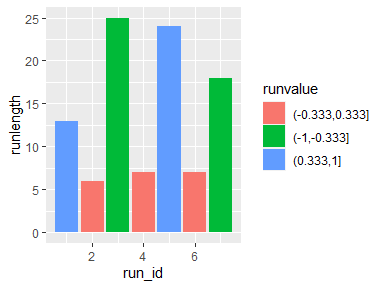# Statistics

library(ggh4x)

# Theoretical densities

One of the more useful tools for inspecting your data is to view the density of datapoints. There are great ways of doing this, such as histograms and kernel density estimates (KDEs). However, sometimes you might wonder how the density of your data compares to the density of a theoretical distribution, such as a normal or Poisson distribution. The stat_theodensity() function estimates the necessary parameters for a range of distributions and calculates the probability density for continuous distributions or probability mass for discrete distributions. The function uses maximum likelihood procedures through the fitdistrplus package.

## Continuous distributions

Plotting continuous distributions is straightforward enough. You just tell stat_theodensity() what distribution you’d like to fit. It automatically performs the fitting for groups separately, as shown in the example below where we artificially split up the faithful data.

df <- faithful
df$group <- ifelse(df$eruptions > 3, "High", "Low")

ggplot(df, aes(eruptions, colour = group)) +
stat_theodensity(distri = "gamma") +
geom_rug()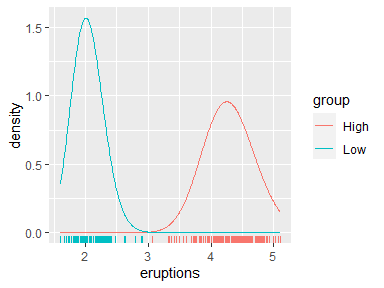We can compare this to kernel density estimates, which are more empirical.

ggplot(df, aes(eruptions, colour = group)) +
stat_theodensity(distri = "gamma",
aes(linetype = "Theoretical")) +
stat_density(aes(linetype = "Kernel Estimates"),
geom = "line", position = "identity") +
geom_rug()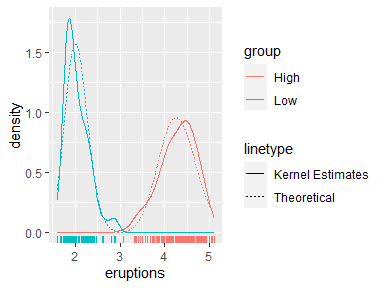There are a few tricky distributions for which there exist no sensible starting values, such as the Student t-distribution and the F-distribution. You would have to provide a sensible-ish starting value for the degrees of freedom for these.

tdist <- data.frame(
x = c(rt(1000, df = 2), rt(1000, df = 4)),
group = rep(LETTERS[1:2], each = 1000)
)

ggplot(tdist, aes(x, colour = group)) +
stat_theodensity(distri = "t", start.arg = list(df = 3))

fdist <- data.frame(
x = c(rf(1000, df1 = 4, df2 = 8), rf(1000, df1 = 8, df2 = 16)),
group = rep(LETTERS[1:2], each = 1000)
)

ggplot(fdist, aes(x, colour = group)) +
stat_theodensity(distri = "f", start.arg = list(df1 = 3, df2 = 3))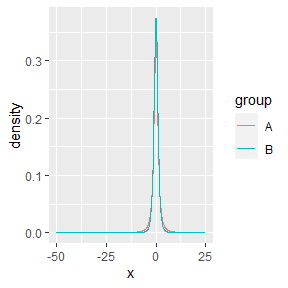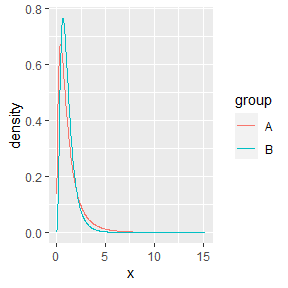## Discrete distributions

The way stat_theodensity() handles discrete distributions is similar to how it handles continuous distributions. The main difference is that discrete distributions require whole number or integer input.

correct <- data.frame(
x = c(rpois(1000, 5), rnbinom(1000, 2, mu = 5)),
group = rep(LETTERS[1:2], each = 1000)
)

incorrect <- correct
# Change a number to non-integer
incorrect$x <- sqrt(2) ggplot(incorrect, aes(x, colour = group)) + stat_theodensity(distri = "nbinom") #> Error in stat_theodensity(): #> ! Problem while computing stat. #> ℹ Error occurred in the 1st layer. #> Caused by error in setup_params(): #> ! A discrete 'nbinom' distribution cannot be fitted to continuous data. ggplot(correct, aes(x, colour = group)) + stat_theodensity(distri = "nbinom")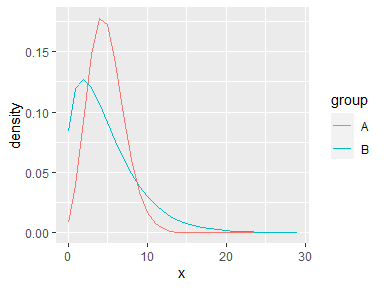A practical difference can be seen above: using simple lines are not very appropriate for discrete distributions, as they imply a continuity that is not there. Instead, one can work with centred steps: ggplot(correct, aes(x, colour = group)) + stat_theodensity(distri = "nbinom", geom = "step", position = position_nudge(x = -0.5))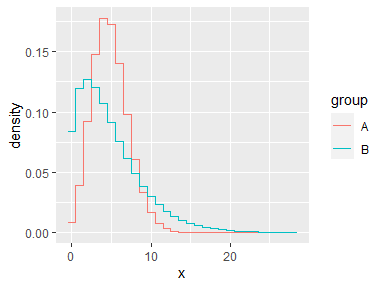Or perhaps most appropriately, you can display the distributions as probability masses through lollipops. ggplot(correct, aes(x, colour = group)) + stat_theodensity(distri = "nbinom", geom = "segment", aes(xend = after_stat(x), yend = 0), alpha = 0.5) + stat_theodensity(distri = "nbinom", geom = "point", aes(xend = after_stat(x), yend = 0)) #> Warning in stat_theodensity(distri = "nbinom", geom = "point", aes(xend = #> after_stat(x), : Ignoring unknown aesthetics: xend and yend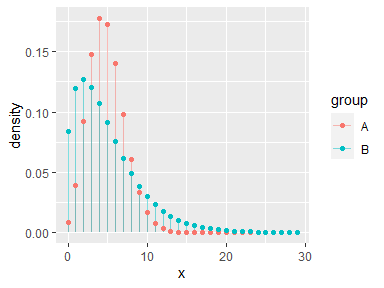## Comparing different distributions Let’s say we are given the task of comparing how well different distributions fit the same data. While we can use more qualitative methods, having a look at the distributions is still a useful tool. We’ll generate some data and see how it works. We’ll fit a normal and Cauchy distribution to the data and plot their densities. set.seed(0) df <- data.frame(x = rnorm(1000, 10, 1/rgamma(1000, 5, 0.2))) ggplot(df, aes(x)) + stat_theodensity(aes(colour = "Normal"), distri = "norm") + stat_theodensity(aes(colour = "Cauchy"), distri = "cauchy") + geom_rug(alpha = 0.1)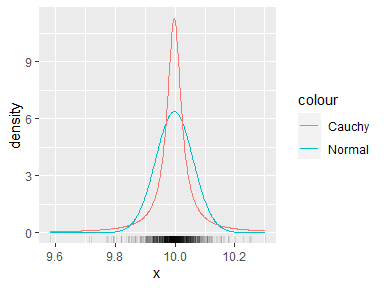From this it is quite hard to see what distribution more appropriately fits the data. To get a clearer view, we can use a histogram instead of a rug plot. The problem though is that by default, histograms work with count data, whereas densities are integrated to sum to 1. ggplot(df, aes(x)) + geom_histogram(binwidth = 0.01, alpha = 0.5) + stat_theodensity(aes(colour = "Normal"), distri = "norm") + stat_theodensity(aes(colour = "Cauchy"), distri = "cauchy")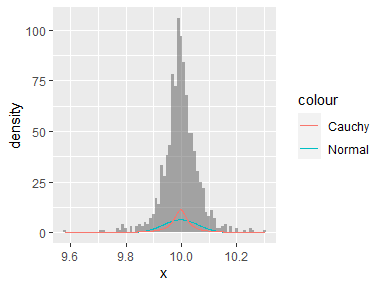There are two possible solutions to this: 1. Scale the histogram to densities. 2. Scale the densities to counts. A nice thing that ggplot2 provides is access to computed variables with the after_stat() function. Luckily, one of the computed variables in histograms already is the density. ggplot(df, aes(x)) + geom_histogram(aes(y = after_stat(density)), binwidth = 0.01, alpha = 0.5) + stat_theodensity(aes(colour = "Normal"), distri = "norm") + stat_theodensity(aes(colour = "Cauchy"), distri = "cauchy")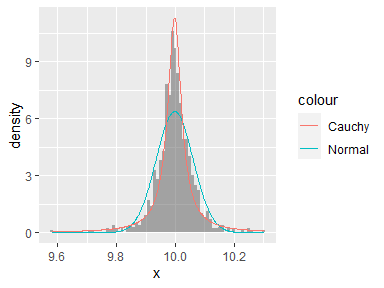Now we can see that probably the Cauchy distribution fits better than the normal distribution. Alternatively, you can also scale the densities to counts. To do this, we must know the binwidth of the histogram. Since different layers in ggplot2 don’t communicate, we have to set these manually. Just like histograms provide the density as computed variable, so too does stat_theodensity() provide a count computed variable, which is the density multiplied by the number of observations. binwidth <- 0.01 ggplot(df, aes(x)) + geom_histogram(alpha = 0.5, binwidth = binwidth) + stat_theodensity(aes(y = after_stat(count * binwidth), colour = "Normal"), distri = "norm") + stat_theodensity(aes(y = after_stat(count * binwidth), colour = "Cauchy"), distri = "cauchy")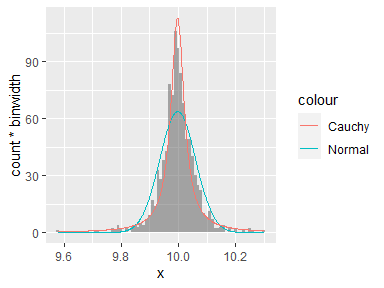# Rolling kernels A rolling kernel is a method that generates a trend line that doesn’t require specifying a model, but is also very bad at extrapolating. It is similar to a rolling window, but data does not need to be equally spaced. An attempt at illustrating the concept you’ll find below.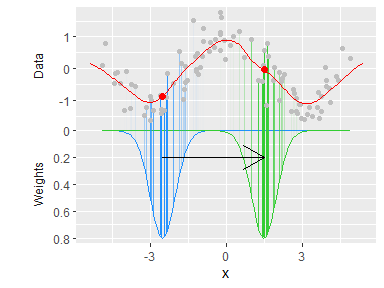For every position on the x-axis, a kernel (above: Gaussian kernel in blue and green) determines the weight of datapoints based on their distance on the x-axis to the position being measured. Then, a weighted mean is calculated that determines the values on the y-axis (red dots). ## Examples Below is an example for a Gaussian kernel on the mpg dataset. ggplot(mpg, aes(displ, hwy, colour = class)) + geom_point() + stat_rollingkernel()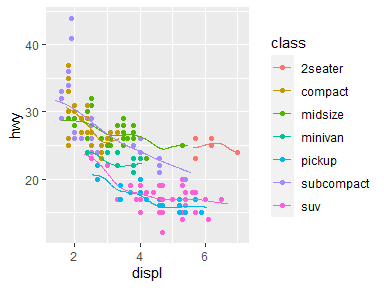It is pretty easy to visualise areas of uncertainty by setting the alpha to scaled weights. This emphasises data-dense areas of the lines. ggplot(mpg, aes(displ, hwy, colour = class)) + geom_point() + stat_rollingkernel(aes(alpha = after_stat(scaled)))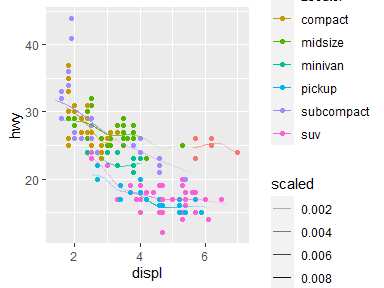## Relation to kernel density estimates It may seem pretty trivial, but using the weights as the y position gives something very similar to kernel density estimates. The defaults for the bandwidth differ slightly, so we exaggerate the similarity by setting them equal here. ggplot(mpg, aes(displ, hwy, colour = class)) + stat_rollingkernel(aes(y = stage(hwy, after_stat = weight), linetype = "Rolling\nKernel"), bw = 0.3) + stat_density(aes(displ, colour = class, y = after_stat(count), linetype = "KDE"), bw = 0.3, inherit.aes = FALSE, geom = "line", position = "identity") + scale_linetype_manual(values = c(2, 1))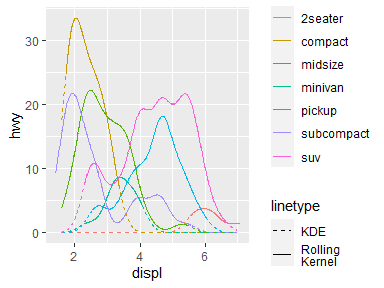## Rolling mean As a final note on this stat, a rolling mean-equivalent can be calculated using the "mean" kernel. This is the same as setting the kernel to "unif", since it uses the uniform distribution as kernel. Typically, this is a bit more blocky than using Gaussian kernels. ggplot(mpg, aes(displ, hwy, colour = class)) + geom_point() + stat_rollingkernel(kernel = "mean", bw = 1)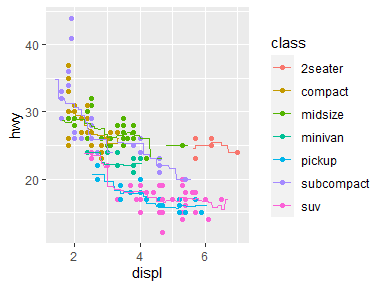# Difference ## Motivation Many people who try to illustrate the difference between two lines might have run into the following problem. In the example below we want to illustrate the difference between the uempmed and psavert variables from the economics dataset, and change the colour of a ribbon depending on which of the variables is larger. Because the groups are inferred from the fill colour, and there are small islands where uempmed > psavert is true, the ribbon will be drawn in an overlapping way. This makes perfect sense for many visualisations, but is an inconvenience when we just want to plot the difference. g <- ggplot(economics, aes(date)) g + geom_ribbon(aes(ymin = pmin(psavert, uempmed), ymax = pmax(psavert, uempmed), fill = uempmed > psavert), alpha = 0.8)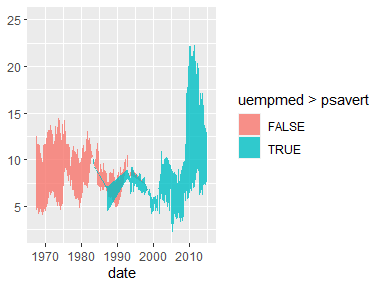To circumvent this inconvenience, stat_difference() uses run-length encoding to re-assign the groups and adds a sign variable to keep track which of the two variables is larger. By default, the fill is populated with the sign variable. g + stat_difference(aes(ymin = psavert, ymax = uempmed), alpha = 0.8)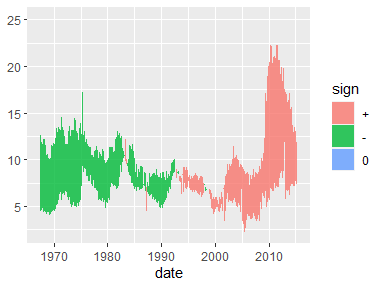## Controlling the fill Because there are 4 dates in the dataset where uempmed == psavert is true, stat_difference() will include a 0 level. The ribbon has no height where this occurs, so you don’t typically see this zero-difference level. The levels argument is included to assert more control over how the sign computed variable will be displayed. In the example below, we remove the 0 level by giving it a NA for sign and telling the fill scale to not translate NAs. g + stat_difference(aes(ymin = psavert, ymax = uempmed), levels = c("More uempmed", "More psavert", NA), alpha = 0.8) + scale_fill_discrete(na.translate = FALSE)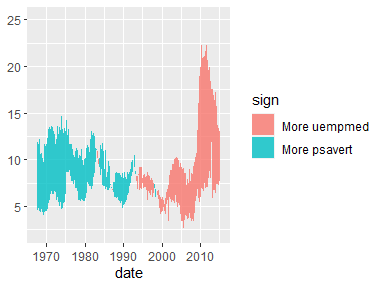## Interpolation An additional nicety of stat_difference() is that it interpolates the cross-over points of lines. It’s not very visible in the densely populated graph above, but we can generate some dummy data to show what we mean. df <- data.frame( x = c(1:4), ymin = c(0, 1, 2, 2.5), ymax = c(2.5, 2, 1, 0.5) ) g <- ggplot(df, aes(x, ymin = ymin, ymax = ymax)) + guides(fill = 'none') + geom_point(aes(y = ymin)) + geom_point(aes(y = ymax)) g + geom_ribbon(aes(fill = ymax < ymin)) + ggtitle("Plain ribbon") g + stat_difference() + ggtitle("stat_difference()")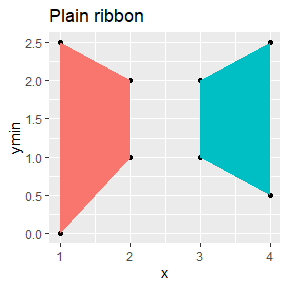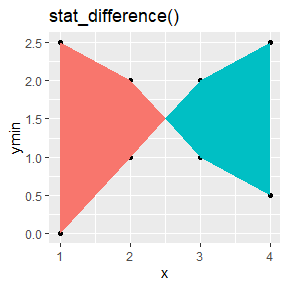# Function X, Y Sometimes, you just want to calculate a simple function on the x- and y-positions of your data by group. That is where stat_funxy() comes in. It takes two functions as arguments, one to be applied to the x-coordinates and one to be applied to the y-coordinates. The primary limitation of this stat is that you cannot use functions that are supposed to work on the x- and y-positions simultaneously. For example, it is pretty easy to combine range and mean to construct range indicators. df <- faithful df$group <- ifelse(df$eruptions > 3, "High", "Low") ggplot(df, aes(waiting, eruptions, group = group)) + geom_point() + stat_funxy(aes(colour = group), funx = range, funy = mean, geom = "line", arrow = arrow(ends = "both"))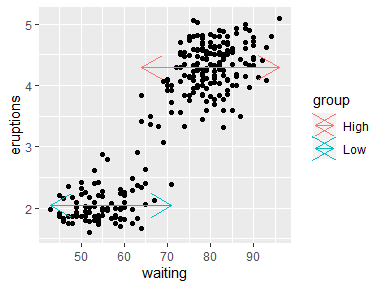## Centroids and midpoints There are also two variations on stat_funxy() and that are stat_centroid() and stat_midpoint(). While the default function arguments in stat_funxy() do nothing, the default for stat_centroid() is to take the means of x- and y-positions and stat_midpoint() takes the mean of the range. Under the hood, these are still stat_funxy(), but have default functions. The centroid and midpoint stats are convenient to label groups, for example. ggplot(df, aes(waiting, eruptions, group = group)) + geom_point() + stat_centroid(aes(label = "Centroid"), colour = "dodgerblue", geom = "label") + stat_midpoint(aes(label = "Midpoint"), colour = "limegreen", geom = "label")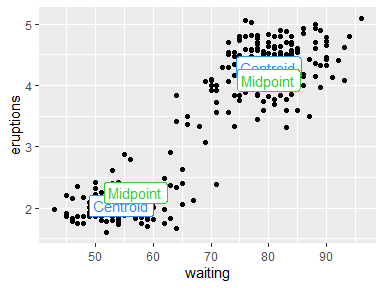## Cropping data While for the general case the data should be cropped to the lengths of the function outputs, you can change this behaviour by setting crop_other = FALSE. This might be convenient when you might have other aesthetics that you care about, in the same group. Not cropping other data probably only makes sense if the functions you provide return a single summary statistic though. ggplot(df, aes(waiting, eruptions, group = group)) + stat_centroid(aes(xend = waiting, yend = eruptions, colour = group), geom = "segment", crop_other = FALSE) + geom_point(size = 0.25)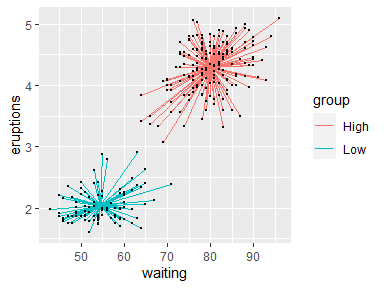# Run length encoding Run length encoding (RLE) is useful as a data compression mechanism, but can also be useful in plotting to check if subsequent conditions are being fulfilled. The default behaviour of stat_rle() is to draw rectangles in the regions where a series of values (a run) have the same value. Let’s say I have the following series: A-A-A-A-B-B-B-C-C-D This series can be compacted by run length encoding, but can also be useful to extract the following properties: run_id run_value run_length start_id end_id 1 A 4 1 4 2 B 3 5 7 3 C 2 8 9 4 D 1 10 10 ## Examples In the example below, we’ll use the cut() function to divide the y-values into three bins, and use the stat_rle() to draw rectangles where datapoints fall into these bins. df <- data.frame( x = seq(0, 10, length.out = 100) ) df$y <- cos(df\$x)

ggplot(df, aes(x, y)) +
stat_rle(aes(label = cut(y, breaks = 3))) +
geom_point()
#> Warning: The following aesthetics were dropped during statistical transformation: y
#> ℹ This can happen when ggplot fails to infer the correct grouping structure in
#>   the data.
#> ℹ Did you forget to specify a group aesthetic or to convert a numerical
#>   variable into a factor?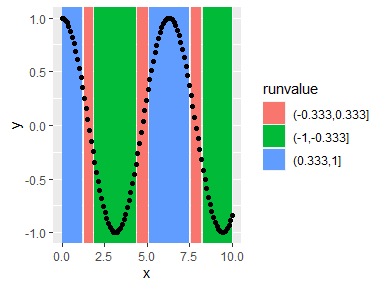This can be made slightly more pleasing by closing gaps between rectangles.

ggplot(df, aes(x, y)) +
stat_rle(aes(label = cut(y, breaks = 3)),
align = "center") +
geom_point()
#> Warning: The following aesthetics were dropped during statistical transformation: y
#> ℹ This can happen when ggplot fails to infer the correct grouping structure in
#>   the data.
#> ℹ Did you forget to specify a group aesthetic or to convert a numerical
#>   variable into a factor?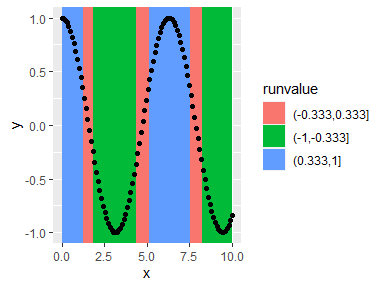## Using computed variables

An alternative use case of stat_rle() is to use the computed variables to describe a series of data. For example, if we’d like to summarise the above graph in just it’s runs, we might be interested in what order the runs are and how long the runs are. If we make use of ggplot2’s after_stat() and stage() functions, we can grab this information from the stat.

ggplot(df) +
stat_rle(aes(stage(x, after_stat = run_id),
after_stat(runlength),
label = cut(y, breaks = 3),
fill = after_stat(runvalue)),
geom = "col")